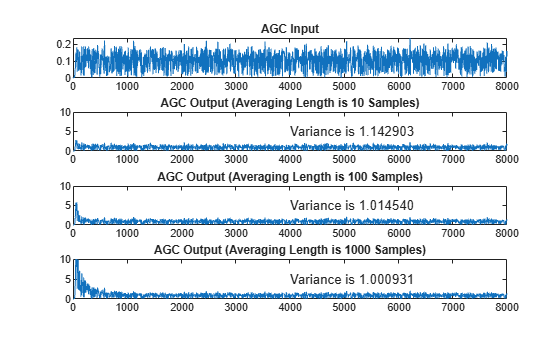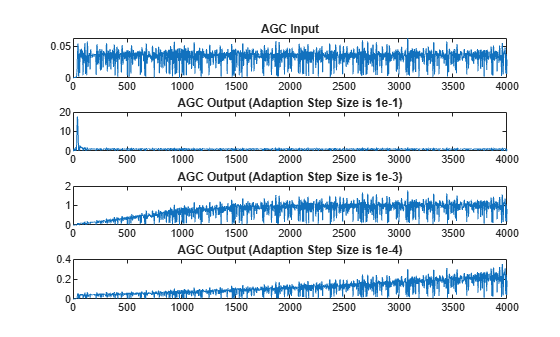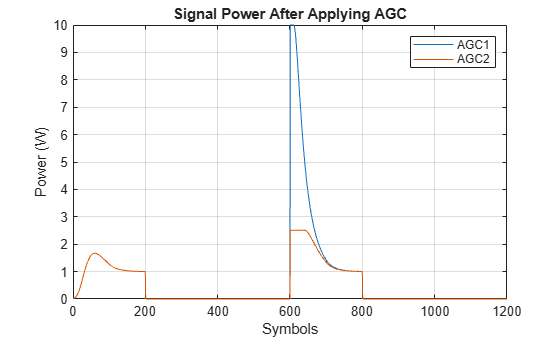# comm.AGC

## Description

The `comm.AGC` System object™ creates an automatic gain controller (AGC) that adaptively adjusts its gain to achieve a constant signal level at the output.

1. Define and set up your automatic gain controller object. See Construction.

2. Call `step` to adaptively adjust gain and achieve a constant signal level at the output according to the properties of `comm.AGC`. The behavior of `step` is specific to each object in the toolbox.

### Note

Starting in R2016b, instead of using the `step` method to perform the operation defined by the System object, you can call the object with arguments, as if it were a function. For example, `y = step(obj,x)` and `y = obj(x)` perform equivalent operations.

## Construction

`H = comm.AGC` creates an AGC System object, `H`, that adaptively adjusts its gain to achieve a constant signal level at the output.

`H = comm.AGC(Name,Value)` creates an AGC object, `H`, with the specified property `Name` set to the specified `Value`. You can specify additional name-value pair arguments in any order as (`Name1`,`Value1`,...,`NameN`,`ValueN`).

## Properties

`AdaptationStepSize`

Specify the step size as a real positive scalar. The default is `0.01`. Increasing the step size permits the AGC to respond more quickly to changes in the input signal level but increases variation in the output signal level during steady-state operation.

`DesiredOutputPower`

Target output power level

Specify the desired output power level as a real positive scalar. The power is measured in Watts referenced to 1 ohm. The default is `1`.

`AveragingLength`

Length of the averaging window

Specify the length of the averaging window in samples as a positive integer scalar. The default is `100`.

### Note

If you use the AGC with higher-order QAM signals, inspect the scatter plot at the output of the AGC during steady-state operation and increase the averaging length if you see frequent gain adjustments. An increase in `AveragingLength` reduces execution speed.

`MaxPowerGain`

Maximum power gain in decibels

Specify the maximum gain of the AGC in decibels as a positive scalar. The default is `60`.

Large gain adjustments can cause clipping when a small input signal power suddenly increases. Use `MaxPowerGain` to avoid large gain adjustments by limiting the gain that the AGC applies to the input signal.

## Methods

 reset Reset internal states of automatic gain controller step Apply adaptive gain to input signal
Common to All System Objects
`release`

Allow System object property value changes

## Examples

collapse all

Apply different AGC averaging lengths to QAM modulated signals. Compare the variance and plot of the signals after AGC is applied.

Initialize three AGC System objects with average window length set to 10, 100, and 1000 samples.

```agc1 = comm.AGC('AveragingLength',10); agc2 = comm.AGC('AveragingLength',100); agc3 = comm.AGC('AveragingLength',1000);```

Generate M-QAM modulated and raised cosine pulse shaped packetized data.

```M = 16; d = randi([0 M-1],1000,1); s = qammod(d,M); x = 0.1*s; pulseShaper = comm.RaisedCosineTransmitFilter; y = awgn(pulseShaper(x),inf);```

Apply AGC.

```r1 = agc1(y); r2 = agc2(y); r3 = agc3(y);```

Plot and compare the signals.

```figure(1) subplot(4,1,1) plot(abs(y)) title('AGC input') subplot(4,1,2) plot(abs(r1)) axis([0 8000 0 10]) title('AGC output (AveragingLength = 10 samples)') text(4000, 5, sprintf('Variance = %f',var(r1(3000:end)))) subplot(4,1,3) plot(abs(r2)) axis([0 8000 0 10]) title('AGC output (AveragingLength = 100 sample)') text(4000, 5, sprintf('Variance = %f',var(r2(3000:end)))) subplot(4,1,4) plot(abs(r3)) axis([0 8000 0 10]) title('AGC output (AveragingLength = 1000 samples)') text(4000, 5, sprintf('Variance = %f',var(r3(3000:end))))```As the averaging length increases the variance at the output of the AGC decreases.

Apply different AGC maximum gain level to QPSK modulated signals. With the maximum gain too small the signal after AGC will not reach desired amplitude. With the maximum gain too large the signal after AGC will overshoot and can saturate resulting in signal loss at the start of received packets. With the maximum gain at the optimal level, the signal after AGC reaches the desired amplitude without signal loss due to saturation. Compare the plot of the signals after AGC is applied.

Initialize three AGC System objects with maximum gain values set to 10 dB, 20 dB, and 30 dB.

```agc1 = comm.AGC('MaxPowerGain', 10); agc2 = comm.AGC('MaxPowerGain', 20); agc3 = comm.AGC('MaxPowerGain', 30); ```

Generate QPSK modulated and raised cosine pulse shaped packetized data.

```M = 4; pktLen = 10000; d = randi([0 M-1],pktLen,1); s = pskmod(d,M,pi/4); x = repmat([zeros(pktLen,1); 0.3*s],3,1); pulseShaper = comm.RaisedCosineTransmitFilter; y = awgn(pulseShaper(x),50); ```

Apply AGC and plot.

```r1 = agc1(y); r2 = agc2(y); r3 = agc3(y); ```

Plot and compare the signals.

```figure(1) subplot(4,1,1) plot(abs(y)) title('AGC input') subplot(4,1,2) plot(abs(r1)) title('AGC output (MaxPowerGain = 10 dB)') subplot(4,1,3) plot(abs(r2)) title('AGC output (MaxPowerGain = 20 dB)') subplot(4,1,4) plot(abs(r3)) title('AGC output (MaxPowerGain = 30 dB)')```This plot compares the signal input to AGC and output from AGC with various maximum gain levels. With the maximum gain set to 10dB, the AGC output cannot reach the desired output power level. With the maximum gain set to 20dB, the AGC output reaches the desired level without saturating. With the maximum gain set to 30dB, the AGC output overshoots risking signal saturation and data loss. Between packets there is only noise in the input signal.

As shown in the plots, with packet transmissions there may be extended periods when no data is received. This results in AGC increasing to the maximum gain setting. If a packet arrives when the AGC gain is too high, the output power overshoots until the AGC can respond to the change in the input power level and reduce its gain.

Apply different AGC step sizes to QPSK modulated signals. Compare the signals after AGC is applied.

Initialize three AGC System objects with step sizes set to 1e-1, 1e-3, and 1e-4.

```agc1 = comm.AGC('AdaptationStepSize',1e-1); agc2 = comm.AGC('AdaptationStepSize',1e-3); agc3 = comm.AGC('AdaptationStepSize',1e-4); ```

Generate QPSK modulated data with raised cosine pulse shaping.

```d = randi([0 3],500,1); s = pskmod(d,4,pi/4); x = 0.1*s; pulseShaper = comm.RaisedCosineTransmitFilter; y = pulseShaper(x); ```

Apply AGC.

```r1 = agc1(y); r2 = agc2(y); r3 = agc3(y); ```

Plot the signal input to AGC and output after various AGC step sizes.

```figure subplot(4,1,1) plot(abs(y)) title('AGC input') subplot(4,1,2) plot(abs(r1)) title('AGC output (AdaptionStepSize = 1e-1)') subplot(4,1,3) plot(abs(r2)) title('AGC output (AdaptionStepSize = 1e-3)') subplot(4,1,4) plot(abs(r3)) title('AGC output (AdaptionStepSize = 1e-4)')```With the step size set to 1e-1, the AGC output overshoot but converges very quickly. With the step size set to 1e-3, the AGC output overshoot disappears and the AGC converges smoothly but more slowly. With the maximum gain set to 1e-4, the AGC takes a very long time to converge.

Modulate and amplify a QPSK signal. Set the received signal amplitude to approximately 1 volt using an AGC. Then plot the output.

Create a QPSK modulated signal using the QPSK System object.

```data = randi([0 3],1000,1); qpsk = comm.QPSKModulator; modData = qpsk(data);```

Attenuate the modulated signal.

`txSig = 0.1*modData;`

Create an AGC System object™ and pass the transmitted signal through it using the `step` function. The AGC adjusts the received signal power to approximately 1 W.

```agc = comm.AGC; rxSig = agc(txSig);```

Plot the signal constellations of the transmit and received signals after the AGC reaches steady-state.

```h = scatterplot(txSig(200:end),1,0,'*'); hold on scatterplot(rxSig(200:end),1,0,'or',h); legend('Input of AGC', 'Output of AGC')```Measure and compare the power of the transmitted and received signals after the AGC reaches a steady state. The power of the transmitted signal is 100 times smaller than the power of the received signal.

```txPower = var(txSig(200:end)); rxPower = var(rxSig(200:end)); [txPower rxPower]```
```ans = 1×2 0.0100 0.9970 ```

Create two AGC System objects™ to adjust the level of the received signal using two different step sizes with identical update periods.

Generate an 8-PSK signal having power equal to 10 W.

```data = randi([0 7],200,1); modData = sqrt(10)*pskmod(data,8,pi/8,'gray');```

Create a pair of raised cosine matched filters with their `Gain` property set so that they have unity output power.

```txfilter = comm.RaisedCosineTransmitFilter('Gain',sqrt(8)); rxfilter = comm.RaisedCosineReceiveFilter('Gain',sqrt(1/8));```

Filter the modulated signal through the raised cosine transmit filter object.

`txSig = txfilter(modData);`

Create two AGC objects to adjust the received signal level. Select a step size of 0.01 and 0.1, respectively. Set the update period to 10.

```agc1 = comm.AGC('AdaptationStepSize',0.01); agc2 = comm.AGC('AdaptationStepSize',0.1);```

Pass the modulated signal through the two AGC objects.

```agcOut1 = agc1(txSig); agcOut2 = agc2(txSig);```

Filter the AGC output signals by using the raised cosine receive filter object.

```rxSig1 = rxfilter(agcOut1); rxSig2 = rxfilter(agcOut2);```

Plot the power of the filtered AGC responses while accounting for the 10 symbol delay through the transmit-receive filter pair.

```plot([abs(rxSig1(11:110)).^2 abs(rxSig2(11:110)).^2]) grid on xlabel('Symbols') ylabel('Power (W)') legend('Step Size 0.01','Step Size 0.1')```The signal with the larger step size converges faster to the AGC target power level of 1 W.

Plot the power of the steady-state filtered AGC signals by including only the last 100 symbols.

```plot((101:200),[abs(rxSig1(101:200)).^2 abs(rxSig2(101:200)).^2]) grid on xlabel('Symbols') ylabel('Power (W)') legend('Step Size 0.01','Step Size 0.1')```The larger AGC step size results in less accurate gain correction.

The reduced accuracy suggests a trade off with the `AdaptationStepSize` property. Larger values result in faster convergence at the expense of less accurate gain control.

Pass attenuated QPSK packet data to two AGCs having different maximum gains. Then display the results.

Create two, 200-symbol QSPK data packets. Transmit the packets over a 1200-symbol frame.

```modData1 = pskmod(randi([0 3],200,1),4,pi/4); modData2 = pskmod(randi([0 3],200,1),4,pi/4); txSig = [modData1; zeros(400,1); modData2; zeros(400,1)];```

Attenuate the transmitted burst signal by 20 dB and plot its power.

```rxSig = 0.1*txSig; rxSigPwr = abs(rxSig).^2; plot(rxSigPwr) grid xlabel('Symbols') ylabel('Power (W)')```Create two AGCs, where `agc1` has a maximum power gain of 30 dB and `agc2` has a maximum power gain of 24 dB.

```agc1 = comm.AGC('MaxPowerGain',30, ... 'AdaptationStepSize',0.02); agc2 = comm.AGC('MaxPowerGain',24, ... 'AdaptationStepSize',0.02);```

Pass the attenuated signal through the AGCs and calculate the output power.

```rxAGC1 = agc1(rxSig); rxAGC2 = agc2(rxSig); pwrAGC1 = abs(rxAGC1).^2; pwrAGC2 = abs(rxAGC2).^2;```

Plot the output power.

```plot([pwrAGC1 pwrAGC2]) legend('AGC1','AGC2') grid xlabel('Symbols') ylabel('Power (W)')```Initially, for the second packet, the `agc1` output signal power is too high because the AGC applied its maximum gain during the period when no data was transmitted. The corresponding `agc2` output signal power does not overshoot the target power level of 1 W by the same amount. It converges to the correct power more quickly due to its smaller maximum gain.

## Algorithms

### Logarithmic Loop AGC

For the logarithmic loop AGC, the output signal is the product of the input signal and the exponential of the loop gain. The error signal is the difference between the reference level and the product of the logarithm of the detector output and the exponential of the loop gain. After multiplying by the step size, the AGC passes the error signal to an integrator.

The logarithmic loop AGC provides good performance for a variety of signal types, including amplitude modulation. Unlike the previous AGC (R2015a and earlier), the detector is applied to the input signal, which results in faster convergence times and increased signal power variation at the detector input. The larger variation is not a problem for floating point systems. A block diagram of the algorithm is shown.Mathematically, the algorithm is summarized as

`$\begin{array}{l}y\left(n\right)=x\left(n\right)\cdot \mathrm{exp}\left(g\left(n-1\right)\right)\\ z\left(n\right)=D\left(x\left(n\right)\right)\cdot \mathrm{exp}\left(2g\left(n-1\right)\right)\\ e\left(n\right)=A-\mathrm{ln}\left(z\left(n\right)\right)\\ g\left(n\right)=g\left(n-1\right)+K\cdot e\left(n\right),\end{array}$`

where

• x represents the input signal.

• y represents the output signal.

• g represents the loop gain.

• D(•) represents the detector function.

• z represents the detector output.

• A represents the reference value.

• e represents the error signal.

• K represents the step size.

### AGC Detector

The AGC detector output, z, computes a square law detector:

`$z\left(m\right)=\frac{1}{N}{\sum }_{n=mN}^{\left(m+1\right)N-1}{|y\left(n\right)|}^{2}\text{\hspace{0.17em}},$`

where N represents the update period.

### AGC Performance Criteria

• Attack time — The duration it takes the AGC to respond to an increase in the input amplitude.

• Decay time — The duration it takes the AGC to respond to a decrease in the input amplitude.

• Gain pumping — The variation in the gain value during steady-state operation.

Increasing the step size decreases the attack time and decay times, but it also increases gain pumping.